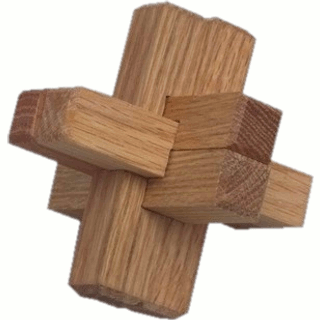6760, Math 21a, Fall 2009
Exhibits page Math 21a 2009, Multivariable Calculus
Triple Cross productIn a homework problem, you had to verify the identity ```a x (b x c) = (a.c)b - (a.b) c ``` Here is how you could verify that fast with Mathematica ```u = {u1, u2, u3}; v = {v1, v2, v3}; w = {w1, w2, w3}; Simplify[ Cross[u, Cross[v, w]] == (u.w) v - (u.v) w ] ``` You can not throw this into wolfram alpha yet to get an answer, but if you copy paste that into Mathematica you should get an answer. You do not have to remember the above identity.